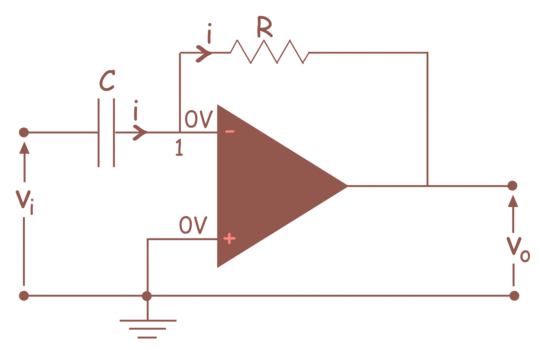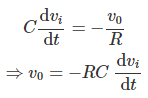# Op Amp Differentiator

Differentiator is an op amp based circuit, whose output signal is proportional to differentiation of input signal.

An op amp differentiator is basically an inverting amplifier with a capacitor of suitable value at its input terminal. The figure below shows the basic circuit diagram of an op amp differentiator.We will first assume that the op amp used here is an ideal op amp. We know that the voltage at both inverting and non inverting terminals of an ideal op amp is same. As the electric potential at non inverting terminal is zero since it is grounded. The electric potential of inverting terminal is also zero, as the opamp is ideal. Because, we know that the electric potential at non – inverting and inverting terminals. It is also known to us that the current entering through inverting and non inverting terminal of an ideal op amp is zero.

Considering, these conditions of an ideal op amp, if we apply Kirchhoff Current Law at node 1 of the op amp differentiator circuit, we get,The above equation shows that the output voltage is the derivative of the input voltage.

Want To Learn Faster? 🎓
Get electrical articles delivered to your inbox every week.
No credit card required—it’s 100% free.